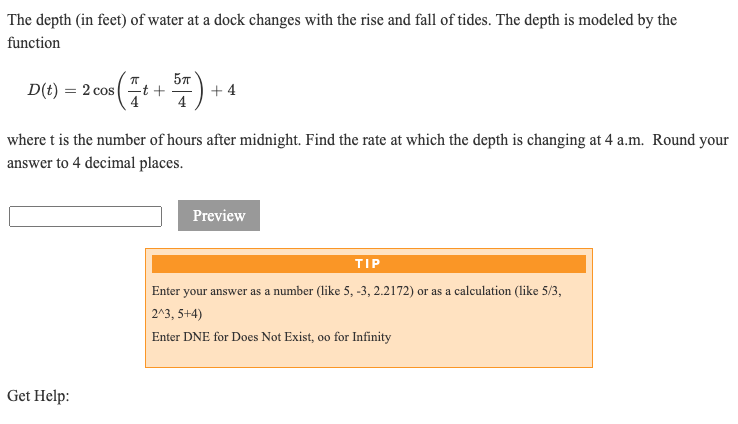# The depth (in feet) of water at a dock changes with the rise and fall of tides. The depth is modeled by the function D(t) = 2 cos + 4 -t + 4 where t is the number of hours after midnight. Find the rate at which the depth is changing at 4 a.m. Round your answer to 4 decimal places. Preview TIP Enter your answer as a number (like 5, -3, 2.2172) or as a calculation (like 5/3, 2^3, 5+4) Enter DNE for Does Not Exist, oo for Infinity

Question

I will appreciate detailed explanation with short comments, so I can do similar exercises.help_outlineImage TranscriptioncloseThe depth (in feet) of water at a dock changes with the rise and fall of tides. The depth is modeled by the function D(t) = 2 cos + 4 -t + 4 where t is the number of hours after midnight. Find the rate at which the depth is changing at 4 a.m. Round your answer to 4 decimal places. Preview TIP Enter your answer as a number (like 5, -3, 2.2172) or as a calculation (like 5/3, 2^3, 5+4) Enter DNE for Does Not Exist, oo for Infinity fullscreen

### Want to see this answer and more?

Experts are waiting 24/7 to provide step-by-step solutions in as fast as 30 minutes!*

*Response times may vary by subject and question complexity. Median response time is 34 minutes for paid subscribers and may be longer for promotional offers.
Tagged in
Math
Calculus

### Applications of Derivative